## Test results

Imaging
We have taken images through the pinhole masks for the SW broad band filters of the Hawaii (J,Js,H,Ks) and the Aladdin (J+Block,H,Ks) arms.

The pinhole mask images have been analysed using sextractor. For each pinhole image we measure the size of the major axis, the position angle of the major axis with the x-axis (measured counter clockwise), and the ratio of the axes (minor/major). The size of the major axis measured by sextractor is defined as the maximum spatial rms of the object profile along any direction. Table 1 gives the range of these measured quantities for each image. Table 2 gives links to the FITS files of these images. Figure 1 is a visualization of the sextractor results, showing the measured ellipses and their positions on the array. The size of the ellipses in this figure is exaggerated.

 pre-move post-move SW imaging tests with pinhole mask filter sigma axis ratio filter sigma axis ratio J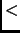0.7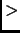0.7 J10.8 Js0.70.7 Js10.8 H0.90.7 H1.10.8 Ks0.80.7 Ks1.10.9 SWLW imaging tests with pinhole mask J+Block10.8 no data H1.10.8 Ks1.10.9

 pre-move post-move SW imaging tests with pinhole mask J FITS image J FITS image Js FITS image Js FITS image H FITS image H FITS image Ks FITS image Ks FITS image SWLW imaging tests with pinhole mask J+Block FITS image no data H FITS image Ks FITS image

For these filters, the image quality with the current collimator position is acceptable. (We have also checked some of the narrow band filters, with the same result).

For the LW imaging (e.g. L & M band filters), the image quality in similar tests is very bad and we have to use the telescope to focus the image (which produces acceptable image quality).

Spectroscopy
For the pinhole mask + continuum spectra we have measured the gaussian fwhm of the spatial profile at 9 positions on the detector - at rows y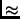780, 320 and 80, and at x=200, 600 and 850 for collimator Position 1 and x=250, 500, 750 for collimator at Position 2. (The slightly different measurement positions for the different collimator positions are of no significance). The results are given in Table 3. If there is a significant spatial distortion it is noted as d (double peaked) or a (asymmetric) in the table. The plots of the spatial profiles at these positions in Figure 2 (SWS) and Figure 3 (LWS) give a better idea of the spatial distortion. Note that in the LWS hot pixels were not removed from the Position 1 data, and this causes additional spikes in a few of the profile plots (for 3.55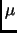m at x,y = (500,750) (750,750) (750,75) and for 4.75m at x,y = (500,75)).

It is clear from the fwhm values and from the plots of the spatial profile that Position 2 (current position) produces better spectroscopic IQ than Position 1.

 Position 1 Position 2 SWS MR 1.25m spatial fwhm spatial fwhm row x=200 x=600 x=850 row x=250 x=500 x=750 785 4.9d 3.8a 4.4a 780 3.5 2.7 2.7 320 3.5 3.5 4.0 320 2.4 2.6 2.8 80 5.4d 4.5a 5.3a 80 4.1 3.6 4.1 SWS MR 1.65m row x=200 x=600 x=850 row x=250 x=500 x=750 785 5.4d 4.5d 5.0a 780 4.1 3.4 3.4 320 4.1 4.1d 4.6a 320 2.9 3.0 3.3 80 6.0a 4.9a 5.8 80 4.6 4.0 4.3 SWS MR 2.16m SWS MR 2.10m row x=200 x=600 x=850 row x=250 x=500 x=750 785 4.9d 4.1d 4.2a 780 4.2d 3.4a 3.1 320 3.5 3.5d 3.8a 320 2.9 3.0 3.3 70 4.3a 4.2a 4.6a 80 4.6d 4.0d 4.3d LWS MR 3.55m row x=200 x=600 x=850 row x=250 x=500 x=750 780 4.0d 3.7a 3.8a 780 3.0 2.8 2.7 315 2.6a 3.4a 3.8d 320 2.4 2.8 3.1 70 4.3a 4.2a 4.6a 80 4.1a 4.0 4.3 LWS MR 4.75m row x=200 x=600 x=850 row x=250 x=500 x=750 780 4.0d 4.0a 4.2a 780 3.0 3.0 3.0 315 3.5a 4.0a 4.3 320 2.8 2.9 3.3 70 4.8a 4.8a 5.2 80 4.1a 3.8 4.2

 Position 1 Position 2 SWS 1.25m FITS image FITS image SWS 1.65m FITS image FITS image SWS 2.16m FITS image FITS image LWS 3.55m FITS imageFITS image LWS 4.75m FITS imageFITS image- no dark subtracted

Sextractor was used to analyse the pinhole mask + arc lamp spectra. Again we measure the size and axis ratio of the pinhole images, and also the position angle (tilt) of each pinhole image. The position of the pinholes in the mask mean that we get 3 good spectra per image (from the central pinoles), at rows 780,320 and 80. In Table 5 we give the range of results per spectrum on the detector. The position angle is the angle between the major axis and the x-axis, measured counter clockwise (i.e. 90 degrees is aligned with the y-axis, that is, no tilt). In science data the absorption and emission lines will be tilted. This tilt should not be confused with the large scale tilt and curvature of the spectra, the tilt of the absorption/emission lines will remain after this large scale tilt has been removed.

 pre-move post-move SWS MR 1.25m row sigma axis ratio tilt row sigma axis ratio tilt 78020.6 50-60 7801.60.6 40-60 32020.6 45-60 3201.60.6 40-60 802.40.6 60-70 8020.5 60-75 SWS MR 1.65m row sigma ellip tilt row sigma ellip tilt 7802.40.6 50-65 7801.80.6 50-70 3202.30.6 40-60 3201.80.6 40-60 802.80.6 60-80 802.20.6 60-70 SWS MR 2.16m SWS MR 2.10m row sigma ellip tilt row sigma ellip tilt 7801.80.6 50-70 no data 3201.60.6 45-60 8020.6 60-70